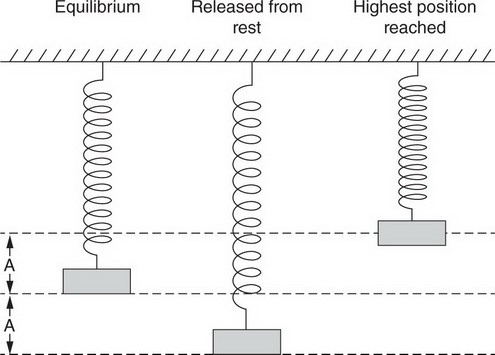# AP Physics 1 Question 111: Answer and Explanation

### Test Information

Question: 111

1.A block is attached to a vertical spring. The block is pulled down a distance A from equilibrium, as shown above, and released from rest. The block moves upward; the highest position above equilibrium reached by the mass is less than A, as shown. When the mass returns downward, how far below the equilibrium position will it reach?

• A. Greater than the distance A below equilibrium
• B. Less than the distance A below equilibrium
• C. Equal to the distance A below equilibrium
• D. No distance—the block will fall only to the equilibrium position.